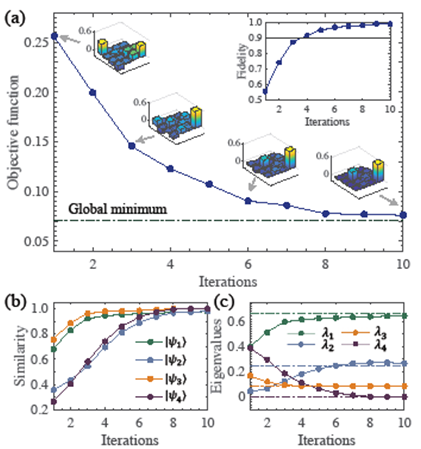HOME / Detail

## SUSTech researchers realize Quantum Principal Component Analysis in experiments

Liangyu CHE     2021-05-08

The Shenzhen Institute of Quantum Science and Engineering (SIQSE) and the Department of Physics at the Southern University of Science and Technology (SUSTech) have recently made important progress in the research of quantum machine learning. Tao Xin, Jun Li, Dawei Lu, and Ying Dong have implemented a quantum principal component analysis algorithm based on parameterized quantum circuits on the nuclear magnetic resonance (NMR) quantum computing platform. Their article was published in the famous journal Physical Review Letters, entitled “Experimental Quantum Principal Component Analysis Via Parameterized Quantum Circuits”.Principal component analysis (PCA) is a common and time-consuming unsupervised learning algorithm in machine learning. This method uses an orthogonal transformation to transform the observed data represented by linearly dependent variables into a few data represented by linearly independent variables. The linearly independent variables are called principal components. In 2014, Lloyd, Mohseni, and Rebentros proposed the quantum principal component analysis (QPCA) algorithm and published their findings in Nature Physics, which can accelerate the classical principal component analysis algorithm exponentially. However, the realization of this algorithm requires a large number of experiment resources, resulting in the lack of experiments to prove the quantum principal component analysis (QPCA).Figure 1. Flow chart of quantum principal component analysis experiment.

Parameterized quantum circuits (PQC) usually consist of fixed gates (such as controlled NOTs) and adjustable gates (such as qubit rotations). PQC formalizes the target problem into a parametric optimization problem and uses a hybrid system of quantum and classical hardware to find approximate solutions. For instance, the variable component eigensolver (VQE) has been used to search for the ground states of the molecule Hamiltonians. Therefore, the research team proposed a new quantum principal component analysis algorithm based on parameterized quantum circuits, which can be implemented experimentally in current quantum platforms.Figure 2. Experimental results of hybrid quantum-classical optimization. (a) Value of objective function as a function of the iteration. Some bar forms of the density matrices are also plotted along with the objective function curve. (b) The similarity of the experimental eigenvectors compared to the ideal ones. (c) Experimental eigenvalues as a function of the iteration and the theoretical eigenvalues are drawn by the dotted horizontal lines.

They further applied the algorithm to face recognition, iteratively optimizing the PQC by using a hybrid quantum-classical control method. The objective function and gradient were measured on a quantum processor, parameters were stored and updated by a classical computer. The QPCA algorithm was implemented in a four-qubit NMR quantum simulator. It is the first time to implement the QPCA algorithm by parameterized quantum circuits, which provides a new way for the theoretical and experimental application research of QPCA.

In this study, Tao Xin, an Assistant Researcher from SUSTech, was the first and corresponding author. Liangyu Che, a Ph.D. student from SUSTech, was the co-first author. Jun Li, an Associate Researcher, Dawei Lu, an Associate Professor from SUSTech, and Ying Dong, a Researcher from the Zhijiang Laboratory, were the co-corresponding authors.

The research was also supported by the Ministry of Science and Technology (MoST), the National Natural Science Foundation of China (NSFC), the Science and Technology Department of Guangdong Province, the Shenzhen Science, Technology and Innovation Commission (SZSTI), and SUSTech.